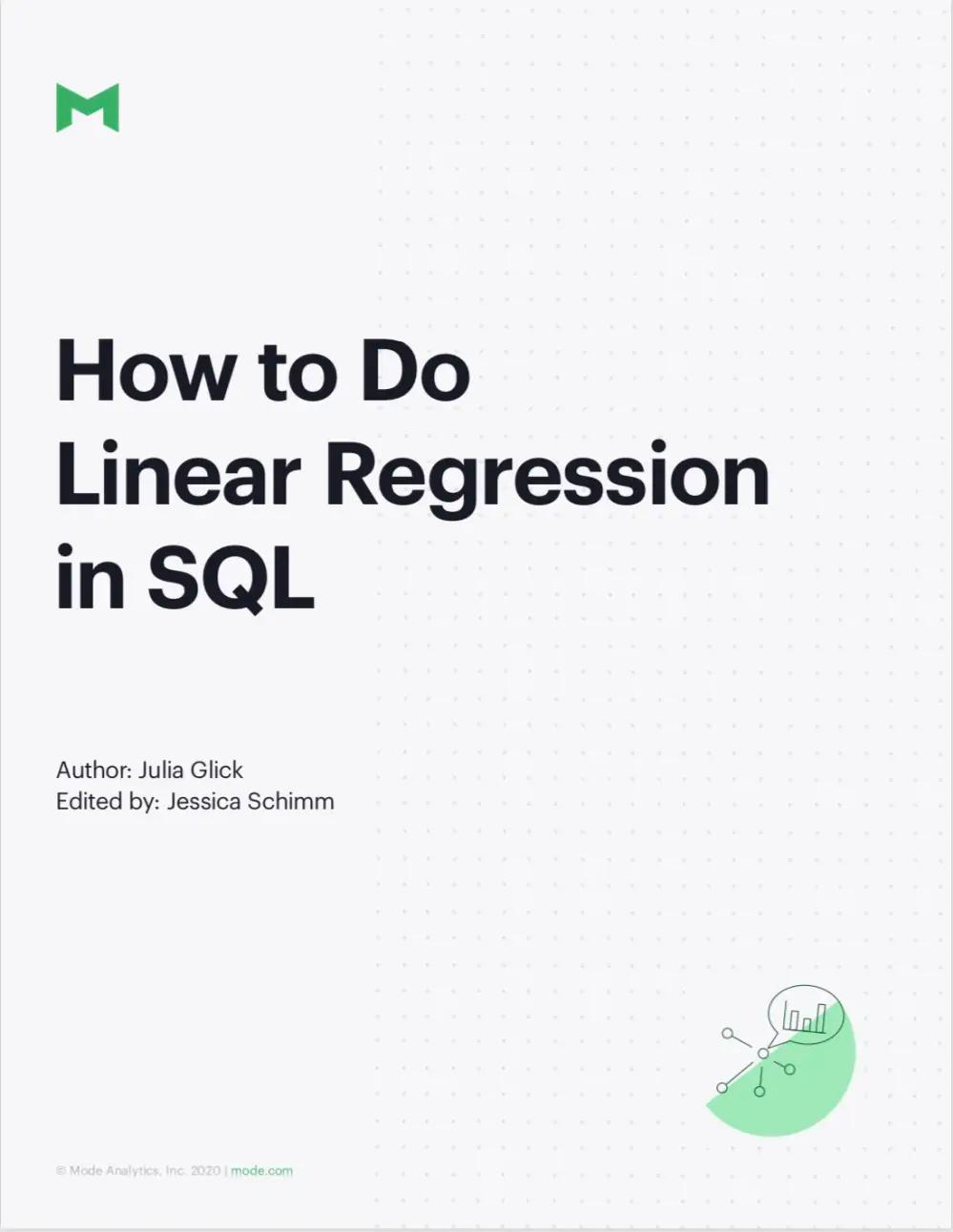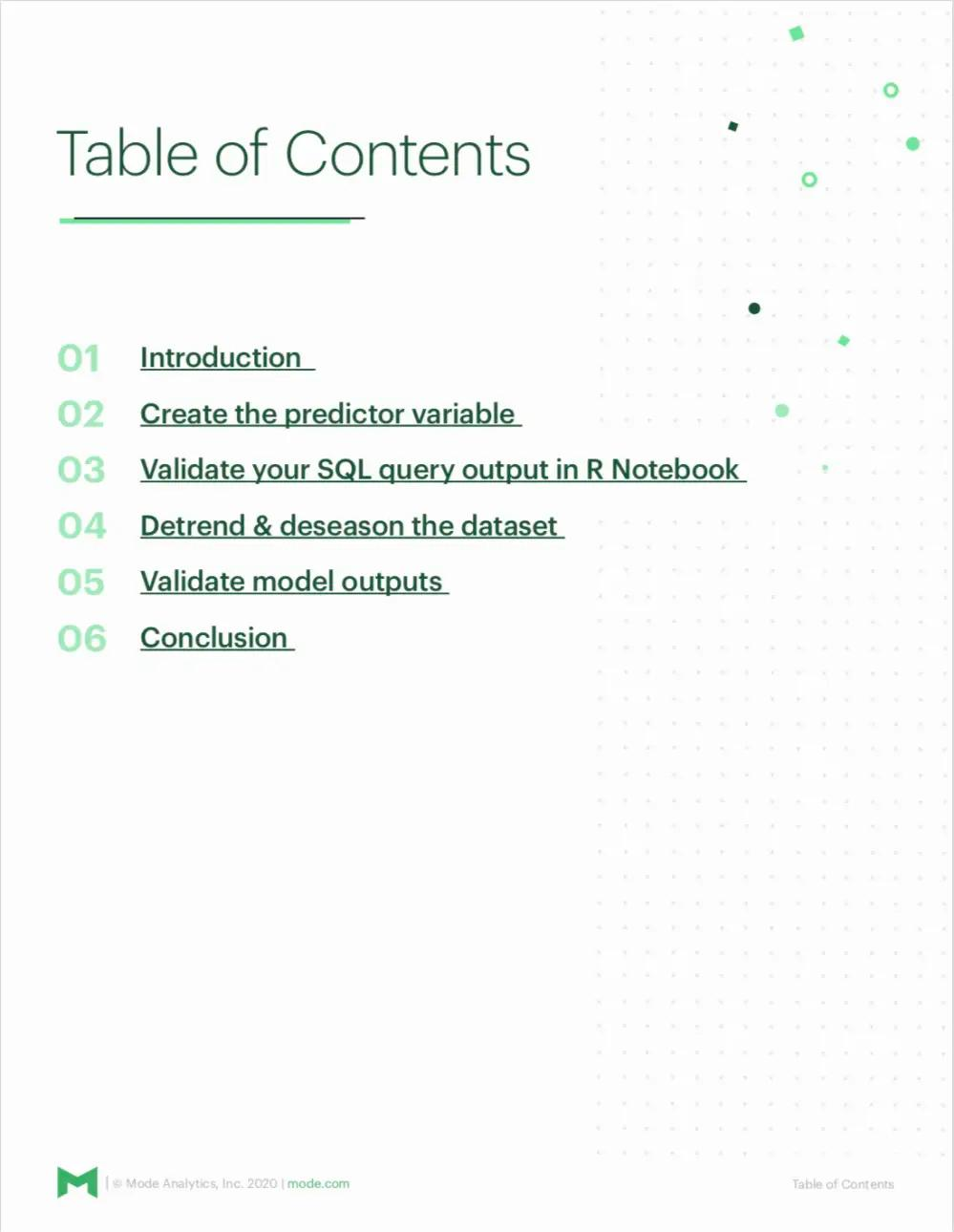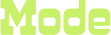How to do linear regression in SQL

### Why we wrote this whitepaper

Linear regression is one of the most powerful tools in a data scientist’s pocket. In this intermediate-advanced tutorial, we’ll show you how you can get a simple regression analysis done in pure SQL with relatively little pain.

In this guide, we will cover:

• Why you would want to do regression in SQL and how to do it in Mode

• How you can test for statistical significance

• How to validate your inputs

• Why simple statistical methods are useful